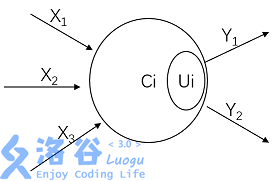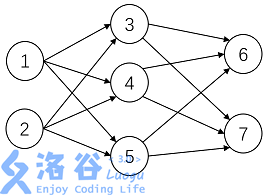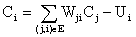# P1038 神经网络

• 4.1K通过
• 11.8K提交
• 题目提供者 CCF_NOI
• 评测方式 云端评测
• 标签 图论 拓扑排序 NOIp提高组 2003
• 难度 普及+/提高
• 时空限制 1000ms / 128MB
• 提示：收藏到任务计划后，可在首页查看。

## 题目背景

人工神经网络（$Artificial Neural Network$）是一种新兴的具有自我学习能力的计算系统，在模式识别、函数逼近及贷款风险评估等诸多领域有广泛的应用。对神经网络的研究一直是当今的热门方向，兰兰同学在自学了一本神经网络的入门书籍后，提出了一个简化模型，他希望你能帮助他用程序检验这个神经网络模型的实用性。

## 题目描述

在兰兰的模型中，神经网络就是一张有向图，图中的节点称为神经元，而且两个神经元之间至多有一条边相连，下图是一个神经元的例子：神经元〔编号为$1$）

图中，$X_1-X_3$是信息输入渠道，$Y_1-Y_2$是信息输出渠道，$C_1$表示神经元目前的状态，$U_i$是阈值，可视为神经元的一个内在参数。

神经元按一定的顺序排列，构成整个神经网络。在兰兰的模型之中，神经网络中的神经元分为几层；称为输入层、输出层，和若干个中间层。每层神经元只向下一层的神经元输出信息，只从上一层神经元接受信息。下图是一个简单的三层神经网络的例子。兰兰规定，$C_i$服从公式：（其中$n$是网络中所有神经元的数目）$C_i=\sum_{(j,i) \in E} W_{ji}C_{j}-U_{i}$

公式中的$W_{ji}$（可能为负值）表示连接$j$号神经元和$i$号神经元的边的权值。当 $C_i$大于$0$时，该神经元处于兴奋状态，否则就处于平静状态。当神经元处于兴奋状态时，下一秒它会向其他神经元传送信号，信号的强度为$C_i$。

如此．在输入层神经元被激发之后，整个网络系统就在信息传输的推动下进行运作。现在，给定一个神经网络，及当前输入层神经元的状态（$C_i$），要求你的程序运算出最后网络输出层的状态。

## 输入输出格式

输入格式：

输入文件第一行是两个整数$n(1 \le n \le 100)$和$p$。接下来$n$行，每行$2$个整数，第$i+1$行是神经元$i$最初状态和其阈值（$U_i$），非输入层的神经元开始时状态必然为$0$。再下面$P$行，每行由$2$个整数$i,j$及$1$个整数$W_{ij}$，表示连接神经元$i,j$的边权值为$W_{ij}$。

输出格式：

输出文件包含若干行，每行有$2$个整数，分别对应一个神经元的编号，及其最后的状态，$2$个整数间以空格分隔。仅输出最后状态大于$0$的输出层神经元状态，并且按照编号由小到大顺序输出。

若输出层的神经元最后状态均为 $0$，则输出 “NULL”。

## 输入输出样例

输入样例#1： 复制
5 6
1 0
1 0
0 1
0 1
0 1
1 3 1
1 4 1
1 5 1
2 3 1
2 4 1
2 5 1

输出样例#1： 复制
3 1
4 1
5 1

提示
标程仅供做题后或实在无思路时参考。
请自觉、自律地使用该功能并请对自己的学习负责。
如果发现恶意抄袭标程，将按照I类违反进行处理。